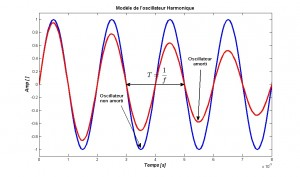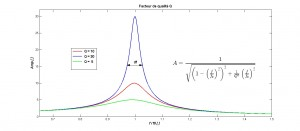Résonique is a portmanteau containing elements from the French words “résonance”, “fréquence sonore” and “énergie mécanique” –  “resonance” “acoustic frequency”, “mechanical energy”. Résonique is continuing the research into sonic horology that began with a few antique clocks that used sonic frequencies (20 Hz – 20 kHz). The guiding principle is the synchronization between the gear train and a mechanical oscillator. The mechanism is using a mechanical power source, or derived energy.

Harmonic oscillator: An oscillator where motion can be described by a sinusoidal function and where frequency depends on its physical characteristics.

Resonator: A device that vibrates at some frequencies through resonance.

Resonance: A physical phenomenon occurring with a system susceptible to specific frequencies. A resonator can cumulate energy if it comes periodically with a frequency close to the system’s natural frequency.

Frequency: The number of occurrences of a repeating event per unit time. It is also referred to as temporal frequency. The period T is the duration of one cycle in a repeating event, so the period is the reciprocal of the frequency. Hertz (Hz) is the unit commonly associated where 5 Hz means 5 oscillations per second. In horology we also use vph (vibration per hour) where 1 Hz is the equivalent of 7200 vph.

Q factor or quality factor: A dimensionless parameter that describes how under-damped an oscillator or resonator is, or equivalently, characterizes a resonator’s bandwidth relative to its center frequency. Higher Q indicates a lower rate of energy loss relative to the stored energy of the oscillator; the oscillations die out more slowly. Sinusoidally driven resonators having higher Q factors resonate with greater amplitudes but have a smaller range of frequencies around that frequency for which they resonate.

Power: The rate at which energy is transferred, used or transformed.

Synchronization: Timekeeping which requires the coordination of events to operate a system in unison.